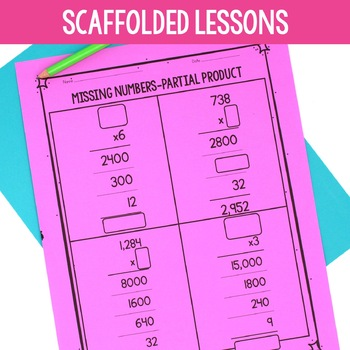# 4th Grade - Multiplication Unit | Distance Learning | Print & Digital4th, Homeschool
Subjects
Standards
Resource Type
Formats Included
• PDF
•Google Apps™
Pages
100 pagesThe Teacher-Author indicated this resource includes assets from Google Workspace (e.g. docs, slides, etc.).

#### Also included in

1. Perfect for guided math and math workshop! This 4th Grade Math Bundle is a year long resource for conceptual math instruction in math workshop or guided math. There are eight units that are each centered around a different mathematical concept: 1. Place Value and Rounding; 2. Addition and Subtractio
\$65.70
\$73.00
Save \$7.30

### Description

Help your students to develop a conceptual understanding of factors, multiples, comparing numbers, and multiplication of large numbers with this four week multiplication unit! Fourth Grade Math Unit 3

This resource includes four weeks of math instruction that is written in an easy to follow format. The lessons are written to give students a solid foundation in finding factors and multiples of numbers, multiplicative comparison, and multiplying 2-digit, 3-digit, and 4-digit numbers by 1-digit numbers, as well as multiplying 2-digit by 2-digit numbers. Students will learn to multiply larger numbers through box models, area models, and partial product. In this unit you will find performance tasks to conceptually teach new skills through the workshop model, as well as math center activities and games for review.

**************************************************************************

What's Included?

-Unit at a Glance

-25 Lesson Plans that include performance tasks

-20 Skill Building Worksheets

- 3 Games (including Multiples Puzzle, Color the Factors, and Number Riddle Task Cards)

***************************************************************************

Check out these other 4th Grade Math Units!

Unit 1 Place Value and Rounding Unit

Unit 2 Addition and Subtraction Unit

Unit 3 Multiplication Unit

Unit 4 Division Unit

Unit 5 Fraction Unit

Unit 6 Decimal Unit

Unit 7 Geometry Unit

Unit 8 Measurement Unit

***************************************************************************

Standards Taught

4.OA.1-Interpret a multiplication equation as a comparison, e.g., interpret 35= 5 × 7 as a statement that 35 is 5 times as many as 7 and 7 times as many as 5. Represent verbal statements of multiplicative comparisons as multiplication equations.

4.OA.2. Multiply or divide to solve word problems involving multiplicative comparison, e.g., by using drawings and equations with a symbol for the unknown number to represent the problem, distinguishing multiplicative comparison from additive comparison

4.OA.4. Find all factor pairs for a whole number in the range 1–100. Recognize that a whole number is a multiple of each of its factors. Determine whether a given whole number in the range 1–100 is a multiple of a given one-digit number. Determine whether a given whole number in the range 1–100 is prime or composite.

4.NBT.5. Multiply a whole number of up to four digits by a one-digit whole number, and multiply two two-digit numbers, using strategies based on place value and the properties of operations. Illustrate and explain the calculation by using equations, rectangular arrays, and/or area models.

Lessons Include:

Pg. 4-5 Lesson 1-What are Multiples

Pg. 6-10 Lesson 2-Multiples Booklet

Pg. 11-13 Lesson 3-What are Factors

Pg. 14-15 Lesson 4-Rolling Factors and Multiples

Pg. 16-17 Lesson 5-Prime and Composite Numbers

Pg. 18-19 Lesson 6-Multiplication as Comparison

Pg. 20-21 Lesson 7-Roll and Compare

Pg. 22-23 Lesson 8-Comparing With 2-Digit Numbers

Pg. 24-25 Lesson 9-Multiplication Scavenger Hunt

Pg. 26-27 Lesson 10-Multiplicative vs. Additive

Pg. 28-30 Lesson 11-Using the Area Model

Pg. 31-32 Lesson 12-Partial Products

Pg. 33-34 Lesson 13-More Area Models

Pg. 35-36 Lesson 14-More Partial Product

Pg. 37-39 Lesson 15-Multiplication Algorithm

Pg. 40-43 Lesson 16-Multiply 2-Digit Numbers by Multiples of Ten

Pg. 44-46 Lesson 17-Expanding Area Models

Pg. 47-49 Lesson 18-Expanding Partial Product

Pg. 50-51 Lesson 19-Roll and Multiply

Pg. 52-53 Lesson 20-Spin and Multiply

Total Pages
100 pages
Included
Teaching Duration
1 month
Report this Resource to TpT
Reported resources will be reviewed by our team. Report this resource to let us know if this resource violates TpT’s content guidelines.

### Standards

to see state-specific standards (only available in the US).
Generate a number or shape pattern that follows a given rule. Identify apparent features of the pattern that were not explicit in the rule itself. For example, given the rule “Add 3” and the starting number 1, generate terms in the resulting sequence and observe that the terms appear to alternate between odd and even numbers. Explain informally why the numbers will continue to alternate in this way.
Find all factor pairs for a whole number in the range 1-100. Recognize that a whole number is a multiple of each of its factors. Determine whether a given whole number in the range 1-100 is a multiple of a given one-digit number. Determine whether a given whole number in the range 1-100 is prime or composite.
Solve multistep word problems posed with whole numbers and having whole-number answers using the four operations, including problems in which remainders must be interpreted. Represent these problems using equations with a letter standing for the unknown quantity. Assess the reasonableness of answers using mental computation and estimation strategies including rounding.
Multiply a whole number of up to four digits by a one-digit whole number, and multiply two two-digit numbers, using strategies based on place value and the properties of operations. Illustrate and explain the calculation by using equations, rectangular arrays, and/or area models.
Recognize that in a multi-digit whole number, a digit in one place represents ten times what it represents in the place to its right. For example, recognize that 700 ÷ 70 = 10 by applying concepts of place value and division.